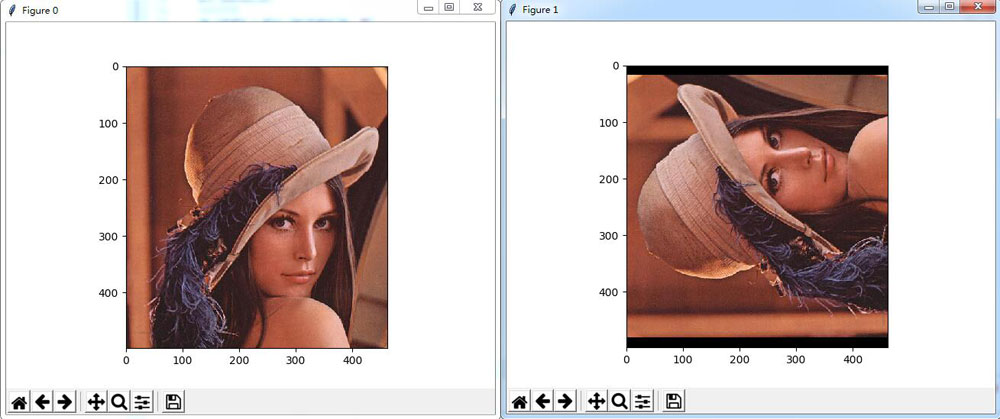# Python图像处理之图像的读取、显示与保存操作【测试可用】

python作为机器学习和图像处理的利器，收到越来越多的推崇，特别是在图像处理领域，越来越多的研究和开发开始转向使用python语言，下面就介绍python图像处理中最基本的操作，即图像的读取显示与保存。

1、使用PIL模块

```# -*- coding:utf-8 -*-
from PIL import Image
import numpy as np
def test_pil():
#读取图像
im = Image.open("lena.jpg")
#显示图像
im.show()
#转换成灰度图像
im_gray = im.convert("L")
im_gray.show()
#保存图像
im_gray.save("image_gray.jpg")
return
test_pil()

```2、使用scipy和matplotlib模块

```# -*- coding:utf-8 -*-
import numpy as np
from scipy import misc
import matplotlib.pyplot as plt
def test_misc():
#读取图像
#显示图像
plt.figure(0)
plt.imshow(im)
#旋转图像
im_rotate = misc.imrotate(im, 90)
plt.figure(1)
plt.imshow(im_rotate)
#保存图像
misc.imsave("lena_rotate.jpg", im_rotate)
plt.show()
return
test_misc()

```#### 您可能感兴趣的文章:

• 本文相关：
• python各类图像库的图片读写方式总结(推荐)
• python使用matplotlib实现的图像读取、切割裁剪功能示例
• python opencv读取png图像转成jpg图像存储的方法
• 浅谈python下tiff图像的读取和保存方法
• python使用pygame绘制图像并保存为图片文件的方法
• python简单图片操作：打开\显示\保存图像方法介绍
• 用python处理图片之打开\显示\保存图像的方法
• python之cv2与图像的载入、显示和保存实例
• numpy 计算两个数组重复程度的方法
• python3实现从指定路径查找文件的方法
• 解决python2.7用pip安装包时出现错误的问题
• 请不要重复犯我在学习python和linux系统上的错误
• 详解python各大聊天系统的屏蔽脏话功能原理
• python下使用scrapy爬取网页内容的实例
• python knn分类算法学习
• python分块读取大数据,避免内存不足的方法
• python3下使用cv2.imwrite存储带有中文路径图片的方法
• python实现图片转字符画的示例代码
• 免责声明 - 关于我们 - 联系我们 - 广告联系 - 友情链接 - 帮助中心 - 频道导航